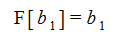# Monoids

Monoids are typically defined as a binary mapping that has an identity element and is associative. In the construction of the Sym application, we have taken an equivalent, but different approach to defining and handling monoids. It may be surprising to see something so fundamental defined in a non-standard way, so the reasoning for taking this approach is explained below.

We will develop a definition of a monoid that is constructed using list objects but is equivalent to the standard definition for a binary mapping. The driver for this approach is that if, for example, addition is defined as a binary function - Add[a, b] - then the expression "w + x + y + z" can be built as any one of 6 different expressions.

The problem that this creates is that we want to be able to pattern match to arbitrary expressions. For example, in doing algebraic manipulations, it's common to want to do things like separating the first term in an expression like "w + x + y + z" from the rest of the expression. Expressed as a pattern match this might look like "a + b..." where the variable "a" is bound to "w" and the variable "b" is bound to all of the other added terms.

Implementing commands like the Left and Right commands, that move terms around in an Add expression is clearly more straight forward to implement if the Add object is just a simple list. Even the most basic highlighting of expressions is much simpler with this approach.

In the Sym application, the monoid property can be applied to objects that have type definitions of the form:

U[] → U

where an object that has type U[] is a named list containing zero or more parameters where each parameter has type U.

Instead of the associativity axiom, in this construction if “F” is a monoid then it has the property thatwhere n , m, and k are any integers greater than or equal to zero. Note that by n = 0, we mean the expression:Conventional binary associativity is recovered through the n = 0, m = 2, k = 1 case:and the n = 1, m = 2, k = 0 case:By appropriate renaming of terms in the these two expressions we can make the right-hand side of both expressions equal, leading to:Identity function:

Consider the case of n = 0, m = 1, k = 0:which implies thatso for any monoid, "F", the one parameter function F[a] is always the identity function.

Identity element:

The n = 0 and m = 0, k = 1 case is:which implies that F[] is the identity element of the function F.

So in this construction, a monoid is defined by a single axiom on a list rather than the two requirements of associativity and an identity in the usual definition.

As a consequence, if a build expression produces a monoid with a single parameter, it will automatically be simplified to just the parameter. So in the example above, we simplify from F[x] to x.